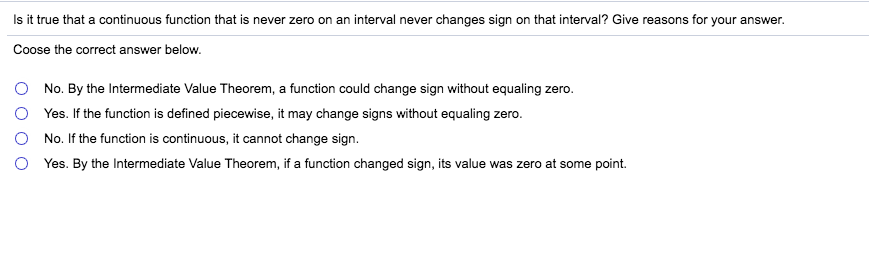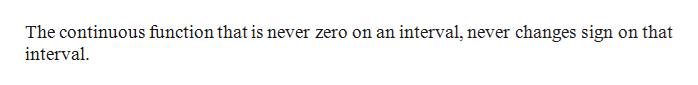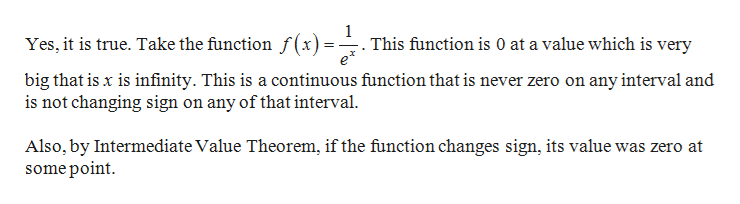Is it true that a continuous function that is never zero on an interval never changes sign on that interval? Give reasons for your answer.Coose the correct answer below.No. By the Intermediate Value Theorem, a function could change sign without equaling zero.Yes. If the function is defined piecewise, it may change signs without equaling zero.No. If the function is continuous, it cannot change signYes. By the Intermediate Value Theorem, if a function changed sign, its value was zero at some point.

Question

Can I get help with this question step by step?help_outlineImage TranscriptioncloseIs it true that a continuous function that is never zero on an interval never changes sign on that interval? Give reasons for your answer. Coose the correct answer below. No. By the Intermediate Value Theorem, a function could change sign without equaling zero. Yes. If the function is defined piecewise, it may change signs without equaling zero. No. If the function is continuous, it cannot change sign Yes. By the Intermediate Value Theorem, if a function changed sign, its value was zero at some point. fullscreen
Step 1

Is it true?help_outlineImage TranscriptioncloseThe continuous function that is never zero on an interval, never changes sign on that interval fullscreen
Step 2help_outlineImage Transcriptionclose1 This function is 0 at a value which is very Yes, it is true. Take the function f (x) e big that is x is infinity. This is a continuous function that is never zero on any interval and is not changing sign on any of that interval Also, by Intermediate Value Theorem, if the function changes sign, its value was zero at some point fullscreen

Want to see the full answer?

See Solution

Want to see this answer and more?

Our solutions are written by experts, many with advanced degrees, and available 24/7

See Solution
Tagged in

Calculus